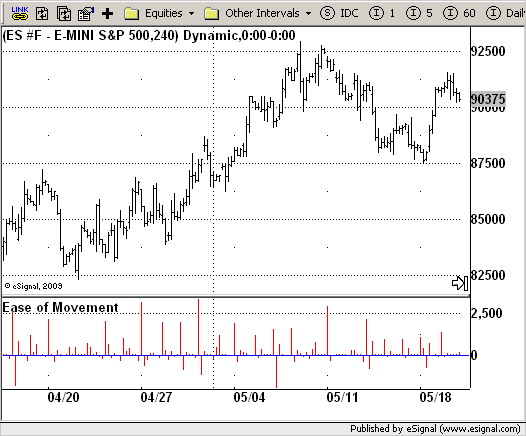# Ease of Movement (EOM)

ICE Data Services -

 EOM.efs EFSLibrary - Discussion Board

File Name: EOM.efs

Description:
Ease of Movement (EOM)

Formula Parameters:

Notes:
This indicator gauges the magnitude of price and volume movement.
The indicator returns both positive and negative values where a
positive value means the market has moved up from yesterday's value
and a negative value means the market has moved down. A large positive
or large negative value indicates a large move in price and/or lighter
volume. A small positive or small negative value indicates a small move
in price and/or heavier volume.
A positive or negative numeric value. A positive value means the market
has moved up from yesterday's value, whereas, a negative value means the
market has moved down.`/*********************************Provided By: eSignal (Copyright c eSignal), a division of Interactive Data Corporation. 2009. All rights reserved. This sample eSignal Formula Script (EFS) is for educational purposes only and may be modified and saved under a new file name. eSignal is not responsible for the functionality once modified. eSignal reserves the right to modify and overwrite this EFS file with each new release.Description: Ease of Movement (EOM) Version: 1.0 05/27/2009 Formula Parameters: Default: Notes: This indicator gauges the magnitude of price and volume movement. The indicator returns both positive and negative values where a positive value means the market has moved up from yesterday's value and a negative value means the market has moved down. A large positive or large negative value indicates a large move in price and/or lighter volume. A small positive or small negative value indicates a small move in price and/or heavier volume. A positive or negative numeric value. A positive value means the market has moved up from yesterday's value, whereas, a negative value means the market has moved down. **********************************/var bInit = false;function preMain() { setStudyTitle("Ease of Movement"); setCursorLabelName("EOM", 0); setDefaultBarFgColor(Color.red,0); addBand(0, PS_SOLID, 1, Color.blue); setPlotType(PLOTTYPE_HISTOGRAM);}var xEOM = null;function main() {var nBarState = getBarState();var nEOM = 0; if (bInit == false) { xEOM = efsInternal("Calc_EOM"); bInit = true; } nEOM = xEOM.getValue(0); if (nEOM == null) return; return nEOM;}var bSecondInit = false;var xHigh = null;var xLow = null;var xVolume = null;var xHalfRange = null;var xMidpointMove = null;function Calc_EOM() {var nRes = 0;var nHigh = 0;var nLow = 0;var nVolume = 0;var nBoxRatio = 0;var nHalfRange = 0;var nMidpointMove = 0;var nMidpointMove_1 = 0; if (bSecondInit == false) { xHigh = high(); xLow = low(); xVolume = volume(); xHalfRange = efsInternal("Calc_HalfRange"); xMidpointMove = mom(1, xHalfRange); bSecondInit = true; } nHigh = xHigh.getValue(0); nLow = xLow.getValue(0); nVolume = xVolume.getValue(0); nHalfRange = xHalfRange.getValue(0); nMidpointMove = xMidpointMove.getValue(0); nMidpointMove_1 = xMidpointMove.getValue(-1); if (nMidpointMove_1 == null) return; if ((nHigh - nLow) != 0) { nBoxRatio = nVolume / (nHigh - nLow); } else { nBoxRatio = 0; } if (nBoxRatio != 0) { nRes = 1000000 * ((nMidpointMove - nMidpointMove_1) / nBoxRatio); } else { nRes = 0; } return nRes;}function Calc_HalfRange() {var nRes = 0; nRes = (high(0) - low(0)) * 0.5; return nRes;} `